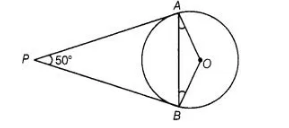# In figure, if PA and PB are tangents

Question:

In figure, if PA and PB are tangents to the circle with centre 0 such that ∠APB = 50°, then ∠OAB is equal to(a) 25°

(b) 30°

(c) 40°

(d) 50°

Solution:

(a) Given, PA and PB are tangent lines.

$\therefore$ $P A=P B$

[since, the length of tangents drawn from an external point to a circle is equal]

$\Rightarrow \quad \angle P B A=\angle P A B=\theta \quad$ [say]

$\ln \triangle P A B, \quad \angle P+\angle A+\angle B=180^{\circ}$

[since, sum of angles of a triangle $=180^{\circ}$ ]

$\Rightarrow \quad 50^{\circ}+\theta+\theta=180^{\circ}$

$\Rightarrow \quad 2 \theta=180^{\circ}-50^{\circ}=130^{\circ}$

$\Rightarrow \quad \theta=65^{\circ}$

Also, $O A \perp P A$

[since, tangent at any point of a circle is perpendicular to the radius through the point of contact]

$\therefore$ $\angle P A O=90^{\circ}$

$\Rightarrow \quad \angle P A B+\angle B A O=90^{\circ}$

$\Rightarrow \quad 65^{\circ}+\angle B A O=90^{\circ}$

$\Rightarrow \quad \angle B A O=90^{\circ}-65^{\circ}=25^{\circ}$